Properties of Metals, Stress-Strain & Elastic Constants - 2

# Properties of Metals, Stress-Strain & Elastic Constants - 2 - Civil Engineering SSC JE (Technical) - Civil Engineering (CE)

Properties of Metals, Stress - Strain and Elastic Constants

ELONGATION OF BARS
1. A bar of uniform cross-sectional area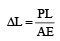2. Non-uniform bar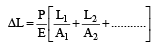3. Tapering bar of circular cross-section whose diameter changes from ‘d1’ at one end to ‘d2’ at the other end.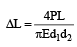where P is the load applied & E is the Young’s modulus of elasticity. L is the length of the specimen.

4. Tapering bar of rectangular cross-section having uniform thickness ‘t’ but width of the bar varies from ‘a’ to ‘b’.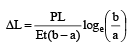5. Bars joined together parallel to each other A structural member composed of two or more elements of different materials to form a parallel arrangement and subjected to axial loading is termed as a compound bar. Such a section is also known as composite section

•  Composite Young’s modulus is given by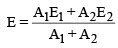Where A1 and E1 are properties of one bar and A2 and E2 are properties of other bar.

6. Elongation due to self weight

(a) For bar of uniform section (Prismatic bar): -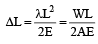Where l = Unit - weight of the material = W/AL
L = Length of the specimen OR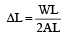W = Total weight of specimen
A = Cross-sectional area.

• Thus, the deformation of the bar under its own weight is half the deformation, if the body is subjected to the direct load equal to the weight of the body.

(b) For bar of tapering section (Conical bar) :

OR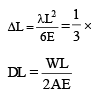Deflection of prismatic bar

7. Bar of Uniform Strength :

• It is possible to maintain uniform stress at all the sections by increasing the area from the lower level to higher level.
• For a bar to have constant strength, the stress at any section, due to external load and the weight of the portion below it should be constant i.e.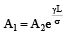Where A1 is the area at upper section where the bar is fixed with immovable support. A2 is area at lower section which is freely hanging.

TEMPERATURE STRESSES

• When a material undergoes change in temperature, its length also changes and if the materials is free to expand or contract, no stresses are developed.

Free expansion in length is given by
ΔL = LaT Where,
L = Length of specimen
α = Coefficient of thermal change
T = Change in temperature

•  If the free expansion or contraction is prevented then stresses will be developed, which is given by σ = αTE
• If the ends of the rod yield by an amount ‘δ ’, in this case the total amount of expansion checked is given by ΔL' = L α T – δ
• It may be noted that thermal stress or temperature stress does not depend upon the cross sectional area.

TEMPERATURE STRESSES IN COMPOSITE SECTIONS
(a) When ends of a compound bar are free to expand :

If the temperature is increased then the metal with greater value of a will be in compression and the other metal will be in tension. On the other hand if the temperature is decreased then the nature of stresses will be change i.e., metal with greater value of a will be in tension and the other metal will be in compression.

Example:- In copper and steel composite section, since coeff. of expansion of copper (αc) is greater than coefficient of expansion of steel (αs) hence free expansion of copper is more than that of steel.

• Let us take actual expansion of composite bar be ‘δ’ then L αc T > δ > L αs T. Therefore steel is in tension and copper is in compression when the temperature is raised.
• Let the σs and σc are stresses in steel and copper. Then for equilibrium of system, Total tension in steel = total compression in copper σsAs = σcAc ...(i)

Also for composite section Actual expansion of steel = Actual expansion of copper, this gives following condition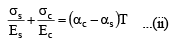Where Es and Ec are modulus of elasticity of steel and copper respectively.
Note : When ends of a compound bar are restrained, then on increasing the temperature metal with greater value of a will be in compression and the other metal will be in tension and vice versa.

(b) When two bars are connected end to end between two fixed supports

• If the temperature is increased both the bars will undergo change in length. But due to the supports, the change in length is restrained. hence both the bars will experience compressive stresses. If the temperature is decreased then both the bars will experience tensile stresses.

Total compressive force = σsAs = σcAc ...(i)
Total extension = 0 Therefore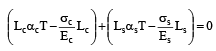ELASTIC CONSTANTS
1. Poisson’s ratio (µ)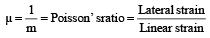The value of m lies between 3 and 4 for most of the metals.
µ = 0.05 to 0.1 for glass = 0.1 to 0.2 for concrete
= 0.25 to 0.42 for metals
= 0.5 for pure rubber (for perfectly plastic body)

2. Volumetric strain ( Îv )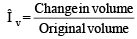• For rectangular bar having, length = l, width = b, and thickness = d. The volumetric strain is given by.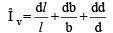or Îv = Îxyz

• For cylindrical rod, having diameter = d.

Volumetric strain ‘ Îv’ is given by
Îv = 2Î+Î l = (2× Diametral strain) + Longitudinal strain

• For spherical body, having diameter = d.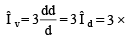Diametral strain

• If a rectangular block is subjected to three normal stresses sx, sy and sz on all its faces, mutually perpendicular to each other in x, y and z directions respectively. Then volumetric strain of the specimen is given by

Îv = Îxyz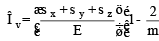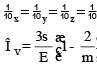3. Shear Modulus

• When a body is subjected to shearing stresses, the shape of the body gets distorted. The measurement of this distortion is done by angle of distortion. Under pure shear the shape of the body gets distorted but the volume remains same.

Modulus of rigidity.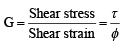Shear modulus is also known as modulus of rigidity.

•  If under the shear, the shear strain is f then the linear strain in the diagonal of the specimen is given by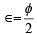linear strain of diagonal is half of the shear strain in the body..
• A shear stress in a given direction cannot exist without a balancing shear stress of equal intensity in a direction at right angles to it. This stress is called complimentary shear stress.

RELATIONSHIP BETWEEN ELASTIC CONSTANTS

Young’s modulus, E = Linear Stress / Linear Strain

Modulus of rigidity, G = Shear stress/ Shear strain

Bulk modulus, K = Direct Stress/ volumetric Strain

Poisson’s ratio, μ = 1/m = Lateral strain/ Linear Strain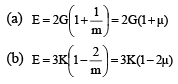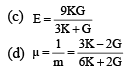STRAIN ENERGY
The strain energy is equal to the work done by the load provided no energy is added or subtracted in the form of heat. It is denoted by U. Thus,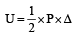stress strain volume = (1/2)
For a prismatic bar,   Δ = PL/ AE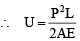• The unit of strain Energy in SI units is Joule (J) which is equal to 1 N-m.
• Resilience is also known as strain energy density. It represents the ability of the material to absorb energy within elastic limit.

It is denoted by ‘u’. Thus,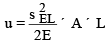When the stress (σ) is equal to proof stress (σf) at the elastic limit, the corresponding resilience is known as proof resilience.  Thus,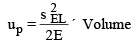• Modulus of Resilience : - Proof Resilience / Volume =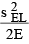• Strain energy of a prismatic bar with varying section is given by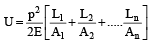• Strain energy of a prismatic bar hanging under its own weight is given by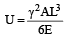• Strain energy of a hanging elastic body due to more than one load cannot be found merely by adding the energies obtained from individual loads. This is because of the fact that strain in such a case is not a linear function but is a quadratic function of the loads.
The document Properties of Metals, Stress-Strain & Elastic Constants - 2 | Civil Engineering SSC JE (Technical) - Civil Engineering (CE) is a part of the Civil Engineering (CE) Course Civil Engineering SSC JE (Technical).
All you need of Civil Engineering (CE) at this link: Civil Engineering (CE)

## FAQs on Properties of Metals, Stress-Strain & Elastic Constants - 2 - Civil Engineering SSC JE (Technical) - Civil Engineering (CE)

 1. What are the properties of metals?Ans. Metals have several distinct properties, including high thermal and electrical conductivity, malleability, ductility, and high melting and boiling points. They are also typically shiny, have high density, and are good conductors of heat and electricity.
 2. What is stress-strain in the context of metals?Ans. Stress-strain refers to the relationship between the force applied to a metal and the resulting deformation or change in shape. Stress is the force per unit area applied to the material, and strain is the resulting change in length or shape of the material. This relationship helps determine the metal's mechanical properties, such as its elasticity, strength, and plasticity.
 3. What are elastic constants in metals?Ans. Elastic constants are parameters that describe a metal's response to an applied stress or strain. They include Young's modulus (modulus of elasticity), which measures the material's stiffness; shear modulus, which measures its resistance to deformation under shear stress; and Poisson's ratio, which relates the lateral and axial strains in a material.
 4. How do metals behave under stress and strain?Ans. When a metal is subjected to stress, it undergoes deformation or changes in shape. Initially, the metal behaves elastically, meaning it returns to its original shape once the stress is removed. However, if the stress exceeds a certain threshold, the metal may undergo plastic deformation, where it permanently changes shape. The behavior of metals under stress and strain is crucial for designing and understanding their mechanical properties.
 5. How do metals' properties affect their applications?Ans. Metals' properties, such as their high thermal and electrical conductivity, malleability, and strength, make them versatile materials in various applications. For example, their conductivity makes them suitable for electrical wiring and heat transfer, their malleability allows for shaping and forming, and their strength makes them useful in structural applications like building construction and manufacturing of vehicles. Understanding the properties of metals helps engineers and designers select the appropriate material for specific applications.

## Civil Engineering SSC JE (Technical)

2 videos|122 docs|55 tests

## Civil Engineering SSC JE (Technical)

2 videos|122 docs|55 tests
Signup to see your scores go up within 7 days! Learn & Practice with 1000+ FREE Notes, Videos & Tests.
10M+ students study on EduRev
Track your progress, build streaks, highlight & save important lessons and more!(Scan QR code)
Related Searches

,

,

,

,

,

,

,

,

,

,

,

,

,

,

,

,

,

,

,

,

,

,

,

,

;